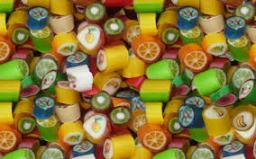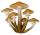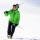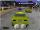# Grandmother

Grandmother wants to give the candies to grandchildren that when she gives 5 candy everyone 3 missing and when she gives 4 candies 3 is surplus. How many grandchildren has grandmother and how many candies has?

Correct result:

b =  27
n =  6

#### Solution:

5n-3 = 4n+3
b = 5n-3

5•n-3 = 4•n+3
b = 5•n-3

n = 6
b-5n = -3

b = 27
n = 6

Our linear equations calculator calculates it.We would be pleased if you find an error in the word problem, spelling mistakes, or inaccuracies and send it to us. Thank you!Tips to related online calculators
Do you have a linear equation or system of equations and looking for its solution? Or do you have quadratic equation?

## Next similar math problems:

• Simple equationSolve for x: 3(x + 2) = x - 18
• Fifth of the numberThe fifth of the number is by 24 less than that number. What is the number?
• Unknown numberIdentify unknown number which 1/5 is 40 greater than one tenth of that number.
• EquationSolve the equation: 1/2-2/8 = 1/10; Write the result as a decimal number.
• FactoryIn the factory workers work in three shifts. In the first inning operates half of all employees in the second inning and a third in the third inning 200 employees. How many employees work at the factory?
• MushroomsFor five days, we have collected 410 mushrooms. Interestingly every day we have collected 10 mushrooms more than the preceding day. How many mushrooms we have collected during 4th day?
• Walnutsx walnuts were in the mission. Dano took 1/4 of nuts Michael took 1/8 from the rest and John took 34 nuts. It stayed here 29 nuts. Determine the original number of nuts.
• Apples 2How many apples were originally on the tree, if the first day fell one third, the second day quarter of the rest and on tree remained 45 apples?
• Divisible by 5How many three-digit odd numbers divisible by 5, which are in place ten's number 3?
• Mom and daughterMother is 39 years old. Her daughter is 15 years. For many years will mother be four times older than the daughter?
• CandyPeter had a sachet of candy. He wanted to share with his friends. If he gave them 30 candies, he would have 62 candies. If he gave them 40 candies, he would miss 8 candies. How many friends did Peter have?
• Ski classClass attend 30 boys and some number of girls. Ski training was attended by 28 boys and all the girls, which was 95% of all students. How many girls attend this class? How many percent is that?
• FriendsJames and Jaroslav are friends. Together they had 48 toy cars. But if John took 8 cars from Jaroslav, Jaroslav will be have 1.5 times more toy cars than James. How many toy cars had Jaroslav and James?
• DivisibilityWrite all the integers x divisible by seven and eight at the same time for which the following applies: 100
• RemainderA is an arbitrary integer that gives remainder 1 in the division with 6. B is an arbitrary integer that gives remainder 2 the division by. What makes remainder in division by 3 product of numbers A x B ?
• Stamps and albumsMichail has in three albums stored 170 stamps. In the first album, there are 14 more than in the second and in the second is by 1/5 less than in the third. How many stamps are in the first album?
• ChildrenLess than 20 children is played various games on the yard. They can create a pairs, triso and quartets. How many children were in the yard when Annie came to them?